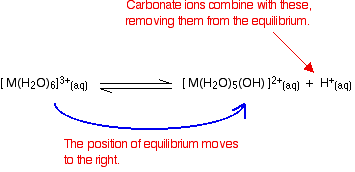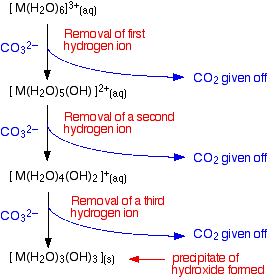# Reactions of the Hexaaqua Ions with Carbonate Ions

•• Contributed by Jim Clark
• Former Head of Chemistry and Head of Science at Truro School in Cornwall

This page describes and explains the reactions between complex ions of the type $$\ce{[M(H2O)6]^{n+}}$$ and carbonate ions from, for example, sodium carbonate solution. There is a difference in the reactions depending on whether the metal at the center of the hexaaqua ion carries a 2+ or a 3+ charge. We need to look at each cases separately.

### 3+ ions reacting with carbonate ions

The 3+ hexaaqua ions are sufficiently acidic to react with carbonate ions to release carbon dioxide gas and precipitate a metal hydroxide.

### The acidity of the 3+ hexaaqua ions

These have the formula $$\ce{[M(H2O)6]^{3+}}$$, and they are fairly acidic. They react with water molecules from the solution:

$\ce{ [M(H2O)6]^{3+} (aq) + H2O <=> [M(H2O)6]^{2+} (aq) + H3O^{+} (aq) }$

They are acting as acids by donating hydrogen ions to water molecules in the solution. Because of the confusing presence of water from two different sources (the ligands and the solution), it is easier to simplify this:

$\ce{ [M(H2O)6]^{3+} (aq) <=> [M(H2O)6]^{2+} (aq) + H^{+} (aq)}$

### Carbonate ions acting as a base

Carbonate ions combine with hydrogen ions in two stages - first to make hydrogencarbonate ions, and then to give carbon dioxide and water.

$\ce{CO3^{2-} (aq) + H^{+} (aq) <=> HCO3^{-} + H2O (l)}$

$\ce{HCO3^{-} (aq) + H^{+} (aq) <=> CO2 (g) + H2O (l)}$

### Reacting carbonate ions with the 3+ hexaaqua ions

Provided the proportions are right, the 3+ hexaaqua ions are sufficiently acidic for the reactions to go all the way to carbon dioxide. There are two possible reactions.

1. Reaction of carbonate ions with the hydroxonium ions (hydrogen ions). According to Le Chatelier's Principle, as the hydrogen ions are removed, the position of equilibrium will move to the right, producing more of the new complex ion.1. Reaction of carbonate ions with the hexaaqua ion. Statistically, there is far more chance of a carbonate ion hitting a hexaaqua metal ion than of hitting a hydrogen ion. It removes a hydrogen ion directly from the hexaaqua complex. If that happens, you get exactly the same new complex ion formed as above. But it doesn't stop there. Carbonate ions keep on removing hydrogen ions from the complex until you end up with a neutral complex. You see that as a precipitate of the metal hydroxide.You can also usefully write the complete change as an overall reaction.

$\ce{ 2 [M(H2O)6]^{3+} (aq) + 3CO3^{2-} -> 2[M(H2O)3(OH)3] (s) + 3CO2 (g) + 3H2O (l)}$

### 2+ ions reacting with carbonate ions

The 2+ hexaaqua ions aren't strongly acidic enough to release carbon dioxide from carbonates. In these cases, you still get a precipitate - but it is a precipitate of what is loosely described as the "metal carbonate".

$\ce{M^{2+} (aq) + CO_3^{2-} (aq) \rightarrow MCO3 (s)}$

## Looking at the ions of specific metals

We'll look at the reactions of three 3+ ions and three 2+ ions. The important ones are the 3+ ions.

### The 3+ ions

#### hexaaquaaluminiumStarting from a colourless solution, you get a white precipitate - but with bubbles of gas as well. The precipitate is identical to the one you get if you add small amounts of either sodium hydroxide or ammonia solutions to a solution of the hexaaquaaluminium ions.

#### hexaaquachromium(III)Again, the precipitate is just the same as if you had added small amounts of either sodium hydroxide or ammonia solution.

#### hexaaquairon(III). . . and again, exactly the same precipitate as if you had added any other base.

Summary

In each case you get a precipitate of the neutral complex - the metal hydroxide. This is exactly the same precipitate that you get if you add small amounts of either sodium hydroxide solution or ammonia solution to solutions of these ions. Bubbles of carbon dioxide are also given off.

### 2+ ions

#### hexaaquacobalt(II)No gas this time - just a precipitate of "cobalt(II) carbonate".

hexaaquacopper(II)Again, there isn't any carbon dioxide - just a precipitate of the "copper(II) carbonate".

hexaaquairon(II)You get a precipitate of the "iron(II) carbonate", but no carbon dioxide.

### Summary

Hexaaqua ions with a 2+ charge aren't sufficiently acidic to liberate carbon dioxide from carbonate ions. Instead you get a precipitate which you can think of as being the metal carbonate.